### Chapter - 4 Basic Geometrical Ideas

Q
##### Basic Geometrical Ideas

Question:

1. Use the figure to name :

(a) Five points

(b) A line

(c) Four rays

(d) Five line segments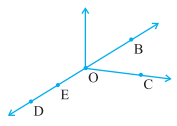Solution: (a) The five points are O, B, C, D, and E.

(b) A line is .

(c) The four rays are .

(d) Five line segments are

2. Name the line given in all possible (twelve) ways, choosing only two letters at a time from the four given.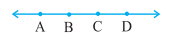Solution: The name of lines are:

3. Use the figure to name :

(a) Line containing point E.

(b) Line passing through A.

(c) Line on which O lies.

(d) Two pairs of intersecting lines.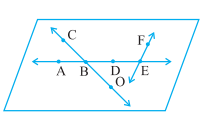Solution: (a) Line  and  contains point E.

(b) Line passing through A is .

(c) Point O lies on the line .

(d)  and  are the two pairs of intersecting lines.

4. How many lines can pass through (a) one given point? (b) two given points?

Solution: (a) Infinite number of lines can pass through the one given point.

(b) One line can pass through the two given points.

5. Draw a rough figure and label suitably in each of the following cases:

(a) Point P lies on .

(b)  and  intersect at M.

(c) Line contains E and F but not D.

(d)  and  meet at O.

Solution: (a)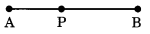(b)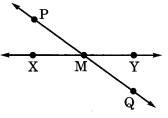(c)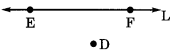(d)6. Consider the following figure of line . Say whether following statements are true or false in context of the given figure.

(a) Q, M, O, N, P are points on the line .

(b) M, O, N are points on a line segment  .

(c) M and N are end points of line segment  .

(d) O and N are end points of line segment  .

(e) M is one of the end points of line segment  .

(f) M is point on ray .

(g) Ray  is different from ray .

(h) Ray  is same as ray  .

(i) Ray  is not opposite to ray .

(j) O is not an initial point of .

(k) N is the initial point of  andSolution: (a) True
(b) True
(c) True
(d) False
(e) False
(f) False
(g) True
(h) False
(i) False
(j) False
(k) True